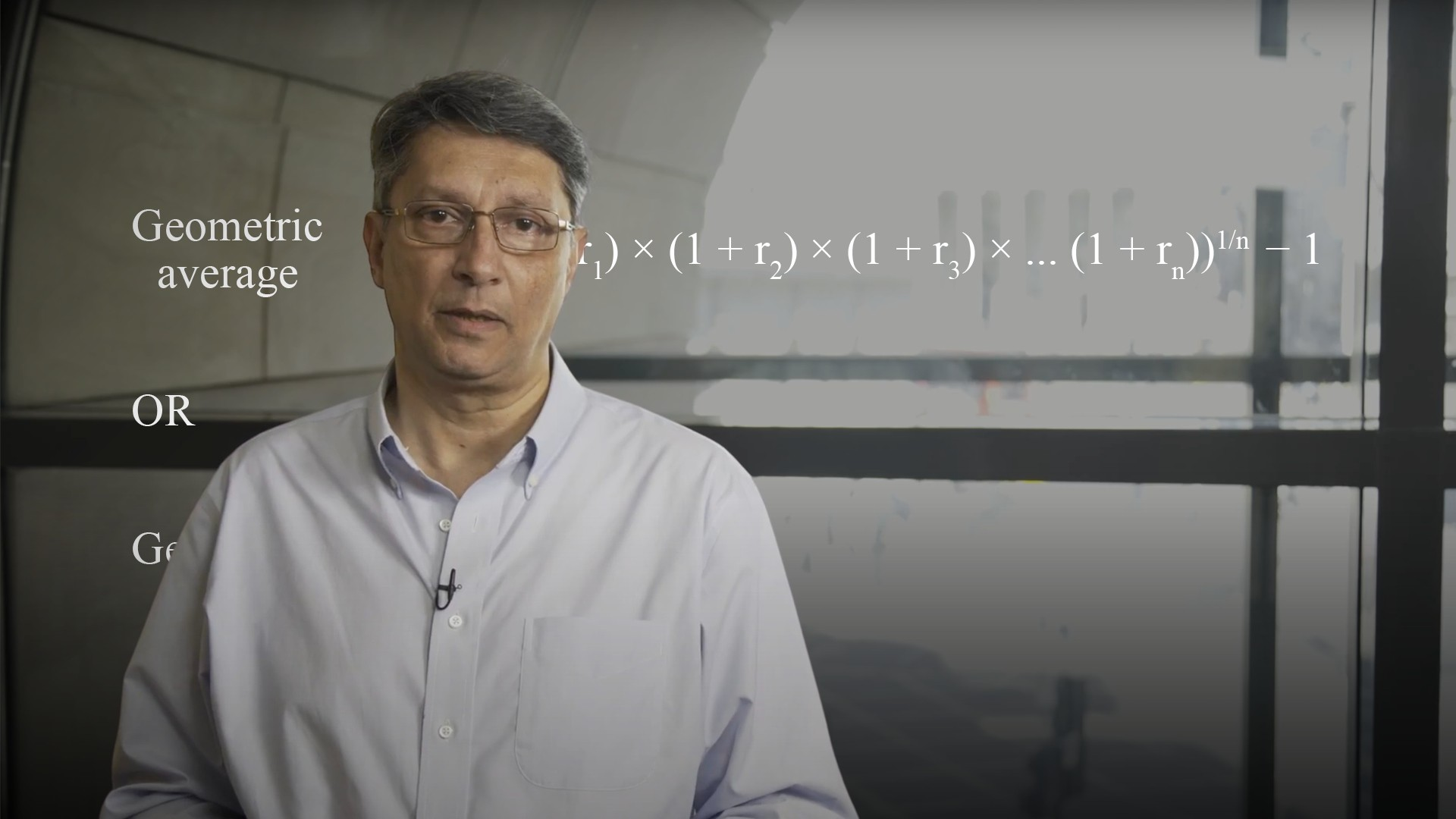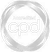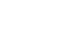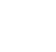# The Geometric Mean### Abdulla Javeri

Abdulla outlines how to use and calculate the geometric average or geometric mean, also referred to as CAGR- the compounded average growth rate.

Financial MathsAbdulla outlines how to use and calculate the geometric average or geometric mean, also referred to as CAGR- the compounded average growth rate.

Speak to an expert

Speak to an expert today to access this and all of the content on our platform.### The Geometric Mean

4 mins 41 secs

Overview

The Geometric Mean is also known as the compounded annual growth rate. The example below shows how to use the geometric mean to calculate the return on a portfolio. It is important to understand that the geometric average should be used instead of the arithmetic mean in the context of a time-series calculation.

Key learning objectives:

• Understand the geometric average

• Identify the situations in which the geometric average is more appropriate than the arithmetic average

## Speak to an expert

Speak to an expert today to access this and all of the content on our platform.

Summary

#### What is the Geometric Mean?

The Geometric Mean is also referred to as CAGR, the compounded average growth rate.

#### How do we calculate the Arithmetic Average?

An example of a portfolio’s return over two years:

In the first year you double your money, and in the following year you lose 50%. If you work out the arithmetic average, you’ll get an annual average growth rate of 25%. That is, 100% minus 50% divided by two. Compounding up at 25% a year should result in a growth in the portfolio value of about 56% over two years, using the formula in the graphic below.

#### Why is the Arithmetic Average inapplicable in the example?

The reality is, there’s been zero growth in the portfolio. If you go from a starting value of 100, and you double your money in year one, you’ll get to 200. If you then lose half of that in year two, you’re back to 100 again.

Clearly we need a different average, enter the geometric average.

#### How do we calculate the Geometric Average?You take 1 plus your annual return for year one, multiply it by 1 plus your annual return for year two, and so on for an ‘x’ amount of years. The next step is to root the result down by the number of years that you’re measuring, take away the 1, and that will give you the geometric average. The reason for using 1 plus the annual returns is to ensure we only have positive numbers in the calculation, you can’t get roots of negative numbers.

#### Which measure is more useful when looking at a time series?

For a time series, the arithmetic average is probably going to be wrong, and thus, we need to use the geometric average if we want to find out some sort of constant compounded rate of return over that time period.

## Speak to an expert

Speak to an expert today to access this and all of the content on our platform.### Abdulla Javeri

Abdulla’s career in the financial markets started in 1990 when he entered the trading floor of the London International Financial Futures Exchange, LIFFE, and qualified as a pit trader in equity and equity index options. In 1996, Abdulla became a trainer for regulatory qualifications and then for non-exam courses, primarily covering all major financial products.

There are no available videos from "Abdulla Javeri"

### Accredited by